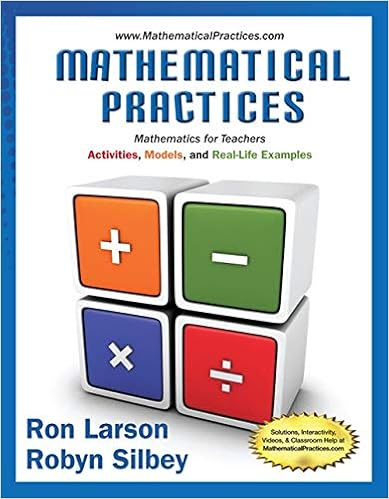# NOTES 09.5 Lagrange Error Bound - Calculus Maximus Notes...

• Notes
• 6
• 100% (1) 1 out of 1 people found this document helpful

This preview shows page 1 - 3 out of 6 pages.

##### We have textbook solutions for you!
The document you are viewing contains questions related to this textbook.The document you are viewing contains questions related to this textbook.
Chapter 6 / Exercise 52
Mathematical Practices, Mathematics for Teachers: Activities, Models, and Real-Life Examples
LarsonExpert Verified
Calculus Maximus Notes 9.5: Lagrange Error Bound Page 1 of 6 §9.5 Lagrange Error Bound Lagrange Form of the Remainder (also called Lagrange Error Bound or Taylor’s Theorem Remainder) Suppose we didn’t have a calculator , but we were interested in the value of sin1 . How could we approximate its value? Eyeball it on the graph? Compare it to 3 sin 3 2 ??? Tangent line approximation? Euler’s method? Taylor polynomial of degree 1 ? Suppose we choose the third or fifth option, after all, polynomials are relatively easy to evaluate. Is there a way, depending on the degree of the polynomial we decide to use, to determine how accurate our approximation is? That is, do we know what remains from our approximation to get the actual value? YES! Yes, we do . . . kind of. When a Taylor polynomial,   n T x , centered at x c is used to approximate a function,   f x , at a value x a near the center, we can express our result as follows: If: Function = Polynomial Approximation + Remainder, Then: Remainder = Function Polynomial Approximation Written mathematically, if: ( ) ( ) ( ) n n f a T a R a Then: ( ) ( ) ( ) n n R a f a P a We define the remainder, ( ) n R a , to be: 1 1 ( ) ( ) ( ) ( 1)! n n n n f z R a T a f a a c n
##### We have textbook solutions for you!
The document you are viewing contains questions related to this textbook.The document you are viewing contains questions related to this textbook.
Chapter 6 / Exercise 52
Mathematical Practices, Mathematics for Teachers: Activities, Models, and Real-Life Examples
LarsonExpert Verified
Calculus Maximus Notes 9.5: Lagrange Error Bound Page 2 of 6 Taylor’s Theorem Historically, the remainder was not due to Taylor but to a French mathematician, Joseph Louis Lagrange (1736-1813), the MVT guy. Historical Note: Up to the time of Mr. Lagrange, there seemed to have been very little interest in determining the error between exact
•••